# 兔年了，利用AI风格化实现剪纸兔、年画兔、烟花兔

## 一、图像风格化简介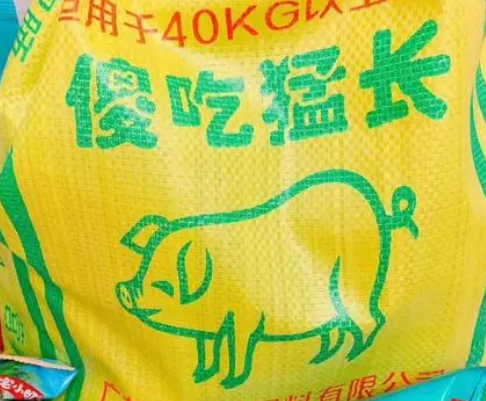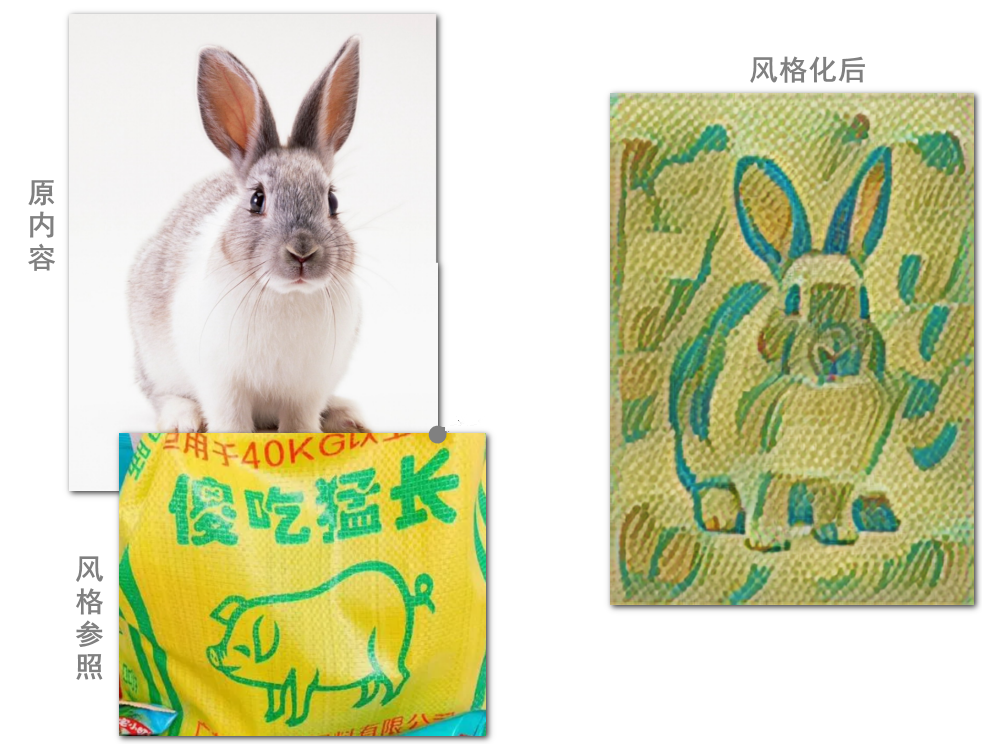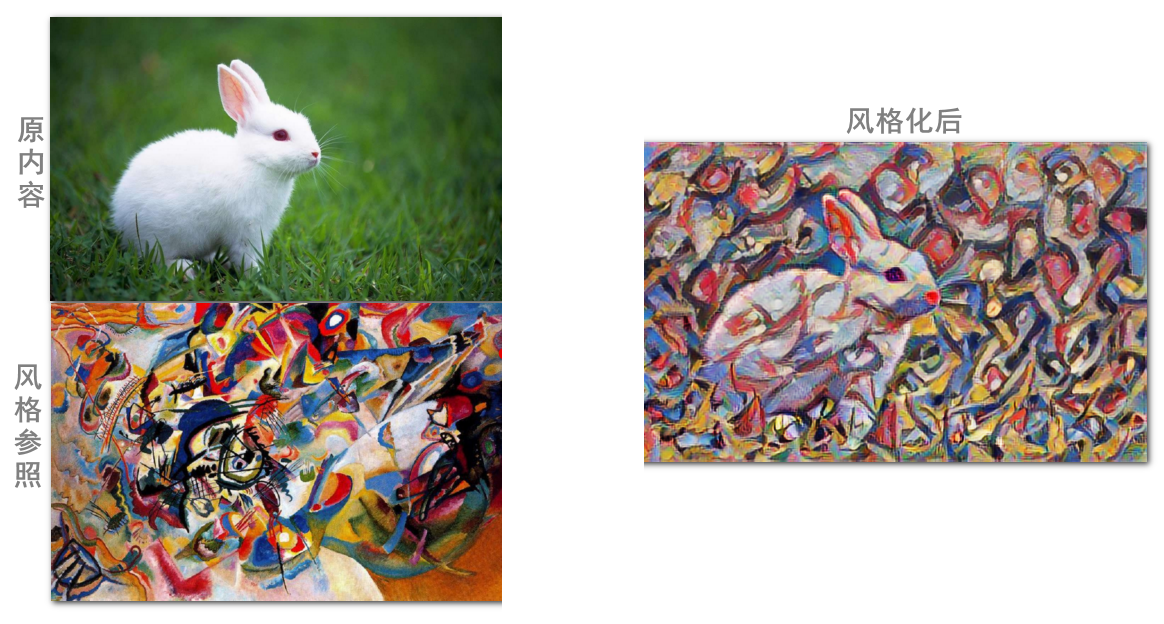## 二、技术实现讲解

### 2.1 TensorFlow Hub库

`TensorFlow` 已经很简单和人性化了。简单到几十行代码，就可以实现数字识别的全流程（我都不好意写这个教程）。

`Hub` 是轮毂的意思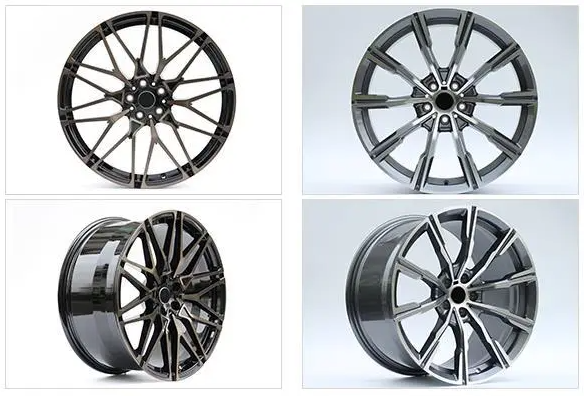### 2.2 加载image-stylization模型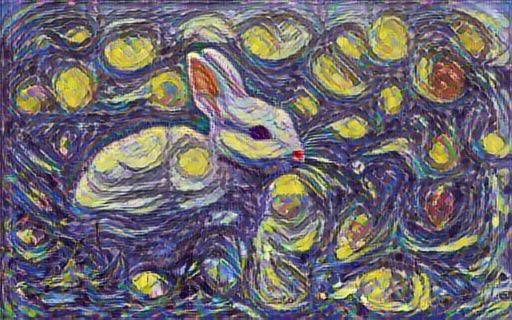``` python

# 导入hub库

import tensorflow_hub as hub

# 将content和style传入

stylized_image = hub_model(content_image, style_image)

# 获取风格化后的图片并打印出来

tensor_to_image(stylized_image) ```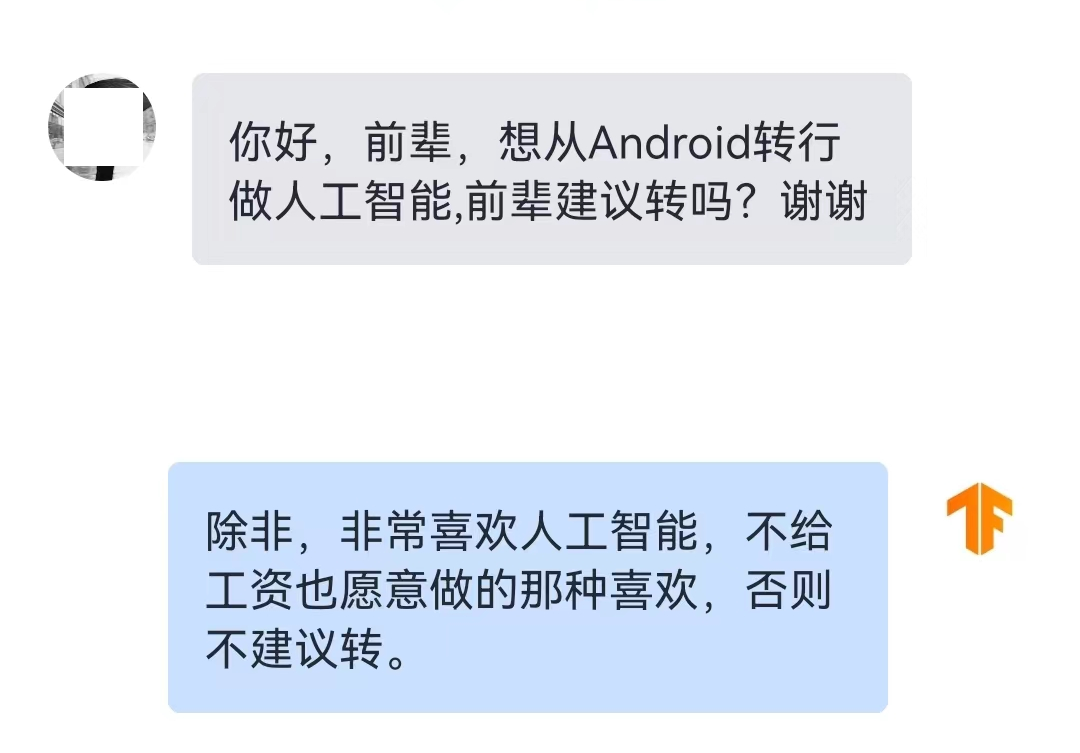### 2.3 输入图片转为tensor格式

`hub_model = hub.load(……)` 是加载模型。我们是加载了图像风格化的模型。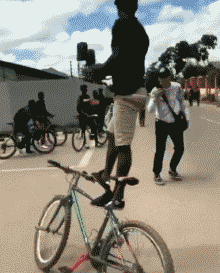`TensorFlow` 中的 `flow` 是什么？这很像《道德经》里的“道”是什么一样。它们只能在自己的语言体系里能说清楚。

``` python import tensorflow as tf

# 根据路径加载图片，并缩小至512像素，转为tensor

max_dim = 512 img = tf.io.read_file(path_to_img) img = tf.image.decode_image(img, channels=3) img = tf.image.convert_image_dtype(img, tf.float32) shape = tf.cast(tf.shape(img)[:-1], tf.float32) long_dim = max(shape) scale = max_dim / long_dim new_shape = tf.cast(shape * scale, tf.int32) img = tf.image.resize(img, new_shape) img = img[tf.newaxis, :] tf_img = tf.constant(img) ```

`read_file` 从路径读入文件。然后通过 `decode_image` 将文件解析成数组。

```python shape=(434, 650, 3), dtype=uint8 array([[[219, 238, 245], ..., [219, 238, 245]], [[219, 238, 245], ..., [219, 238, 245]]])```

`shape=(434, 650, 3)` 说明这是一个三维数组，看数据这是一张650×434像素且具有RGB三个通道的图片。其中的array是具体像素的数值，在某个颜色通道内，255表示纯白，0表示纯黑。

```python shape=(434, 650, 3), dtype=float32 array([[[0.8588236, 0.9333334, 0.9607844], ..., [0.8588236, 0.9333334, 0.9607844]], [[0.8588236, 0.9333334, 0.9607844], ..., [0.8588236, 0.9333334, 0.9607844]]])```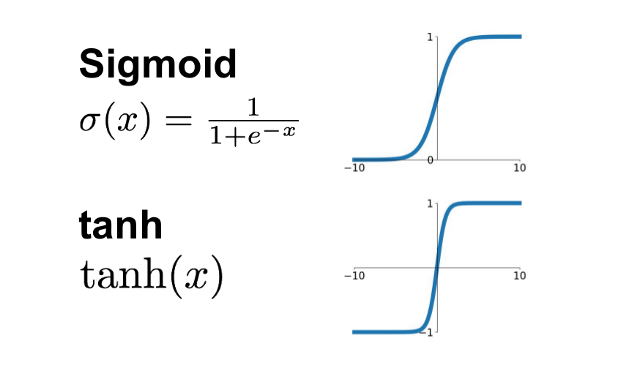```python shape=(341, 512, 3), dtype=float32 array([[[0.8588236, 0.9333334, 0.9607844], ..., [0.8588236, 0.9333334, 0.9607844]], [[0.8588236, 0.9333334, 0.9607844], ..., [0.8588236, 0.9333334, 0.9607844]]])```

`(434, 650, 3)` 代表的是一张图。但是纵观所有算法模型，不管是 `model.fit(train_ds)` 训练阶段，还是 `model.predict(tf_imgs)` 预测阶段。就没有处理单张图片的代码逻辑，全都是批量处理。

```python shape=(1, 341, 512, 3), dtype=float32 array([[[[0.8588236, 0.9333334, 0.9607844], ..., [0.8588236, 0.9333334, 0.9607844]], [[0.8588236, 0.9333334, 0.9607844], ..., [0.8588236, 0.9333334, 0.9607844]]]])```

`shape=(1, 341, 512, 3)` 表示有1张512×341的彩图。那么，这个结构它也可以承载100张这样的图，那时就是`shape=(100, 341, 512, 3)`。这就做到了，以不变应万变。

### 2.4 tensor格式结果转为图片

```python [<tf.Tensor: shape=(1, 320, 512, 3), dtype=float32, numpy= array([[[[0.31562978, 0.47748038, 0.7790847 ], ..., [0.7430198 , 0.733053 , 0.6921962 ]], [[0.76158 , 0.6912774 , 0.5468565 ], ..., [0.69527835, 0.70888966, 0.6492392 ]]]], dtype=float32)>]```

``` python import numpy as np import PIL.Image

tensor = stylized_images tensor = tensor*255 tensor_arr = np.array(tensor, dtype=np.uint8) img_arr = tensor_arr img = PIL.Image.fromarray(img_arr) img.save(n_path) ```

• 第1步：取结果中的第一个 `stylized_images` ，那是 `shape=(1, 320, 512, 3)`
• 第2步：小数转为255色值的整数数组 `tensor*255``np.array(tensor, dtype=np.uint8)`
• 第3步：取出 `shape=(1, 320, 512, 3)` 中的那个1，也就是512×320的那张图。
• 第4步：通过 `fromarray(img_arr)` 加载图片的数组数据，保存为图片文件。

## 三、一切皆可兔图的效果

### 3.1 年画兔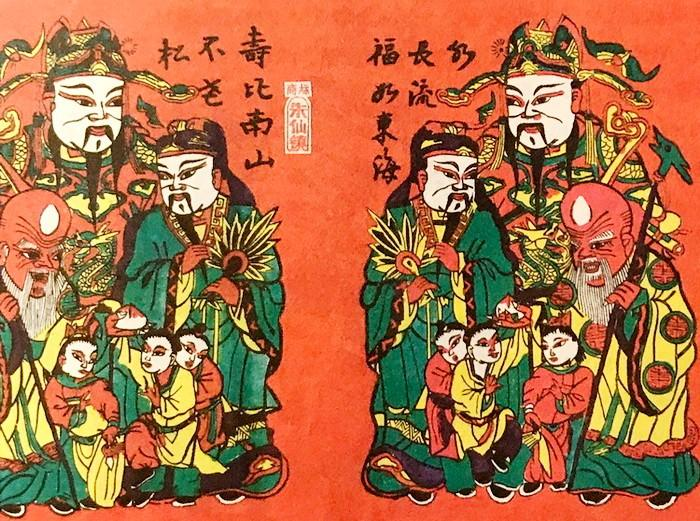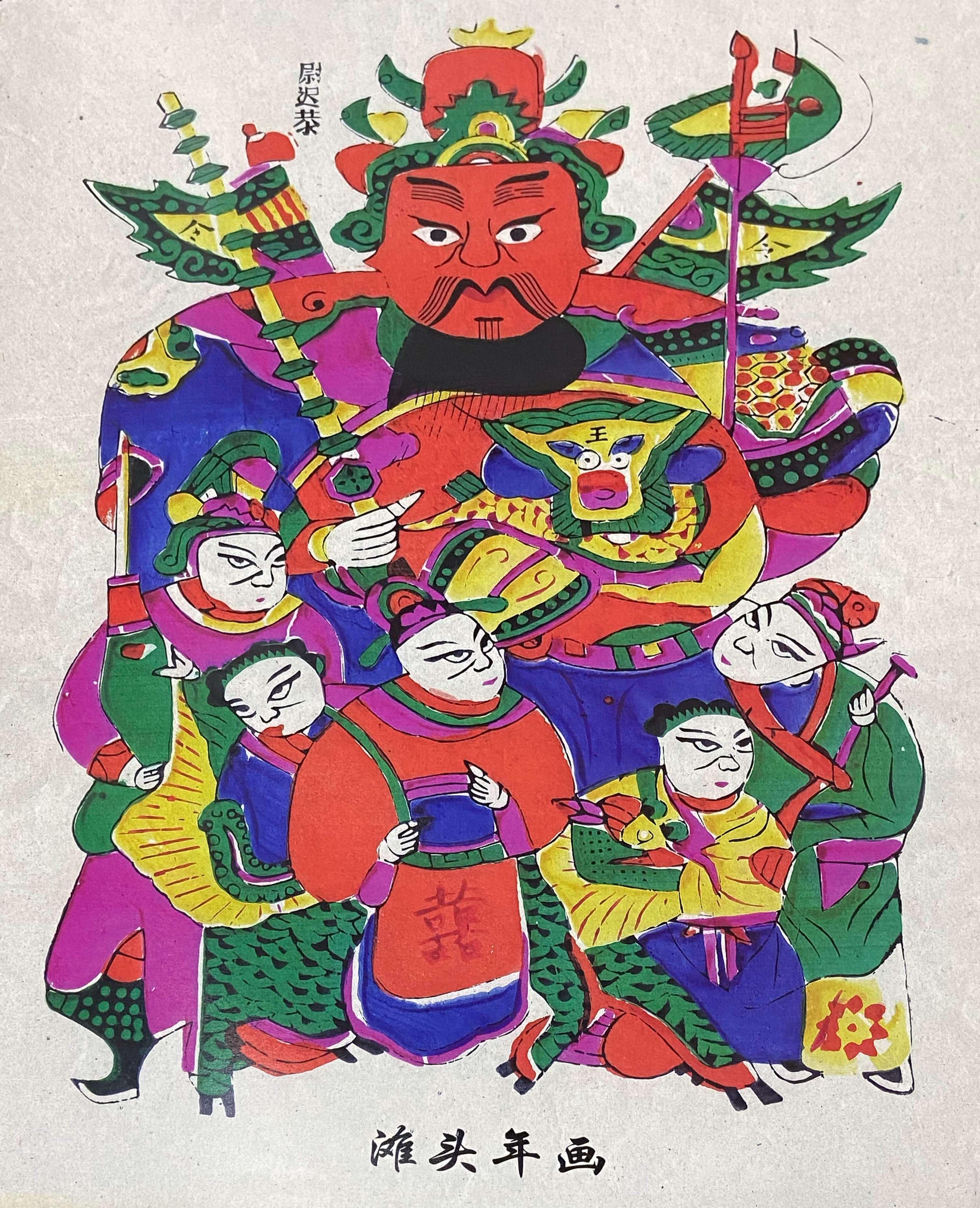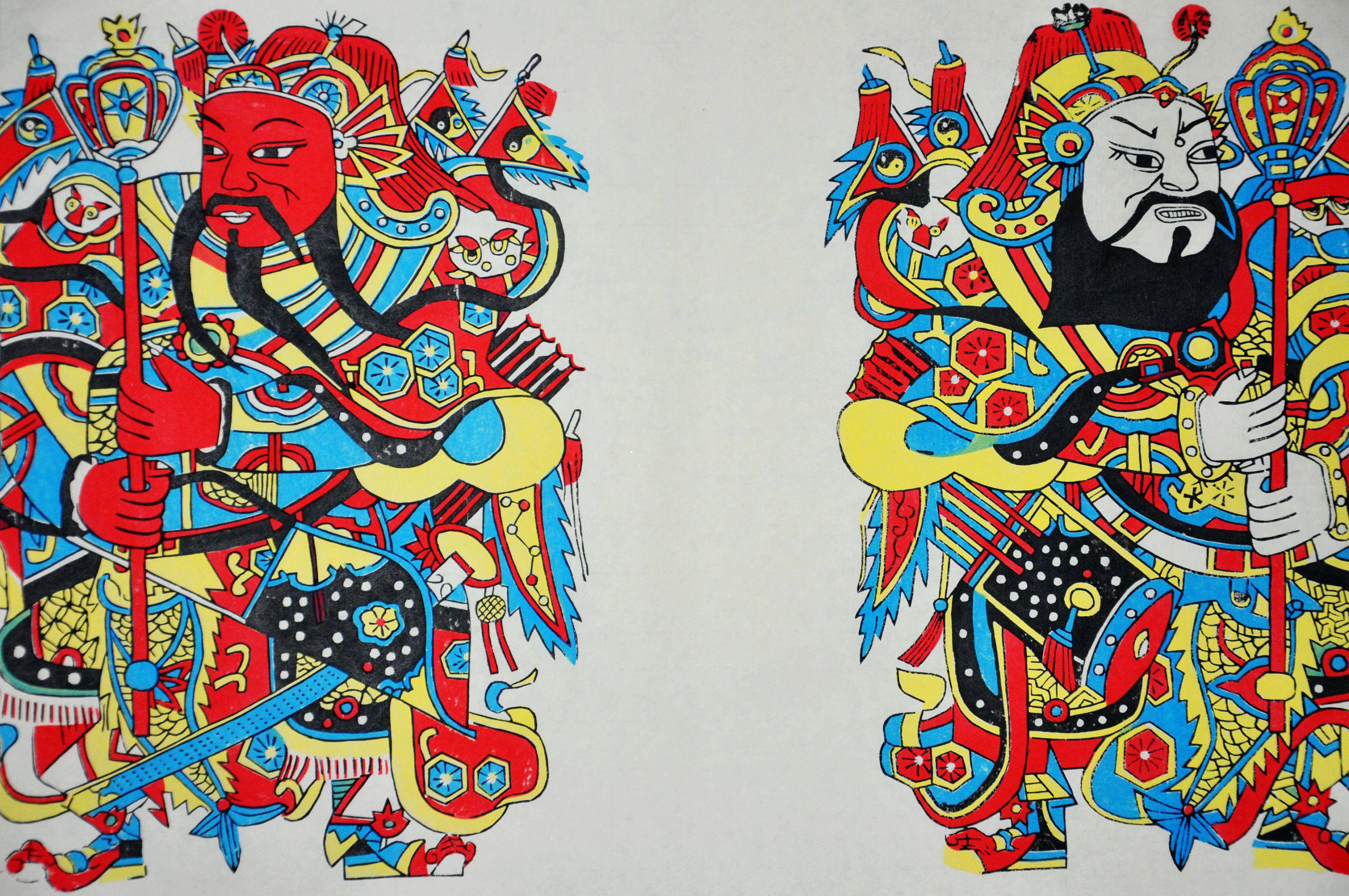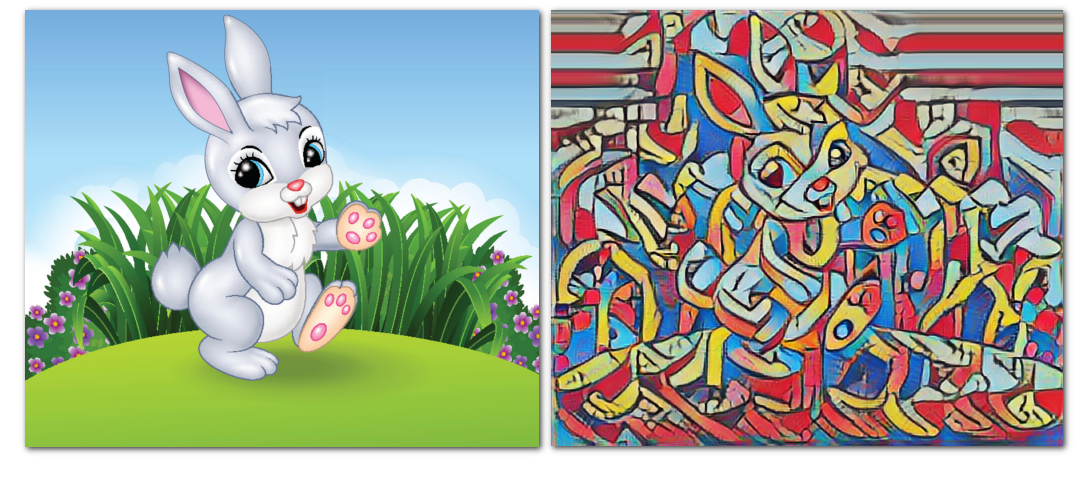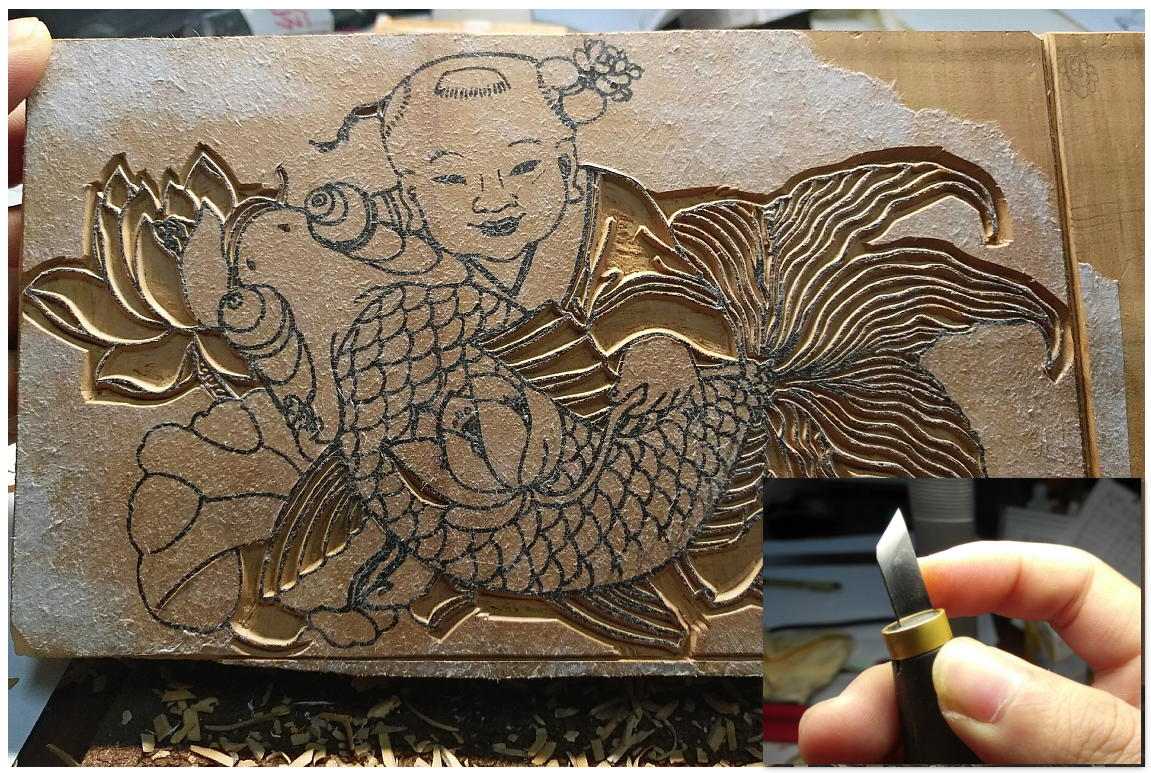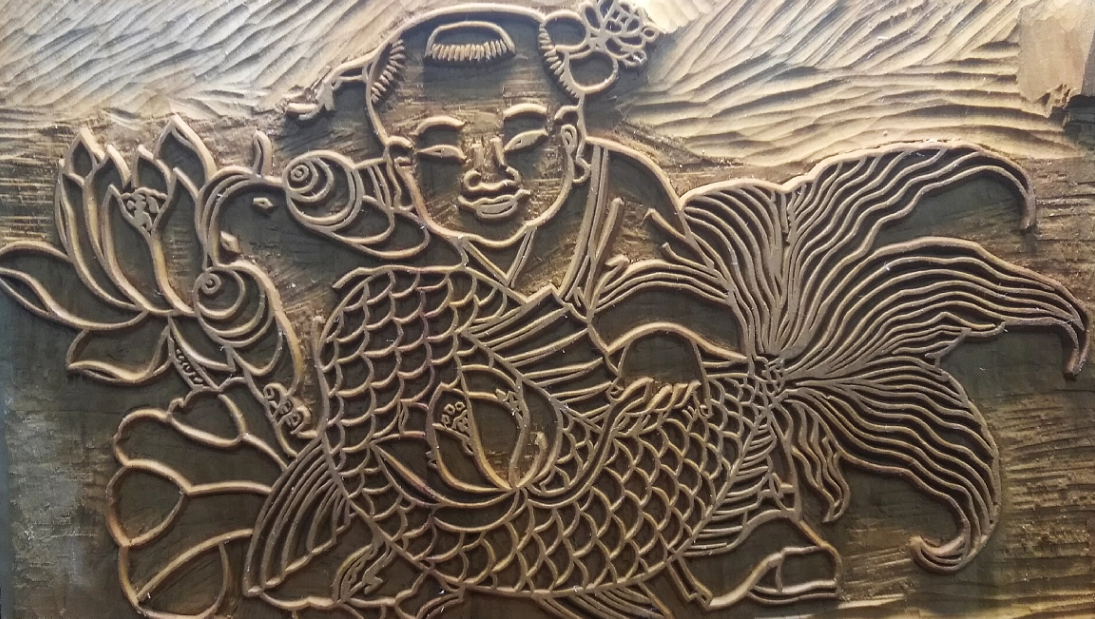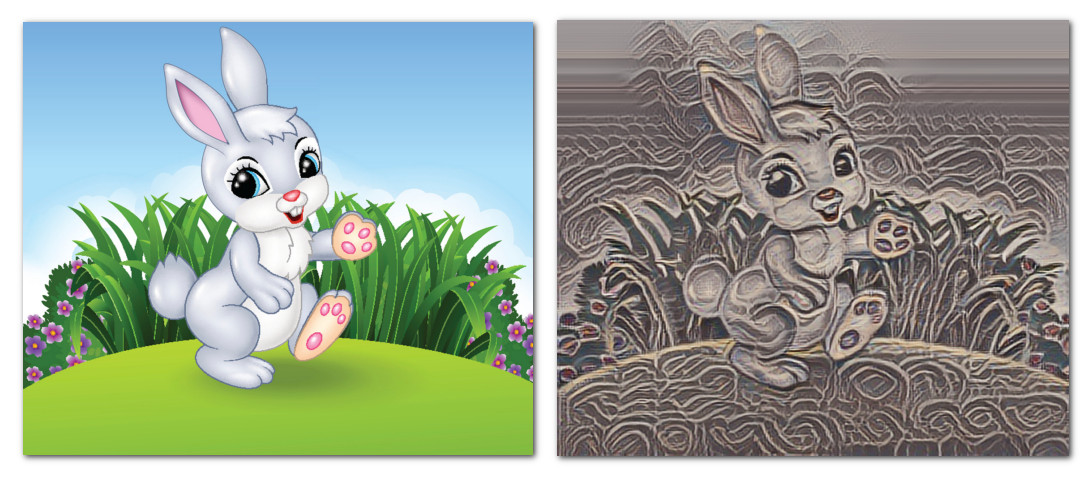### 3.2 剪纸兔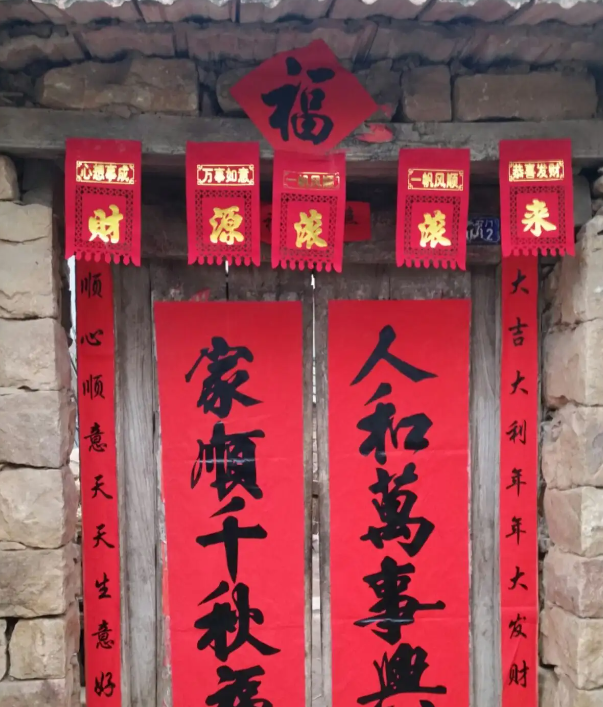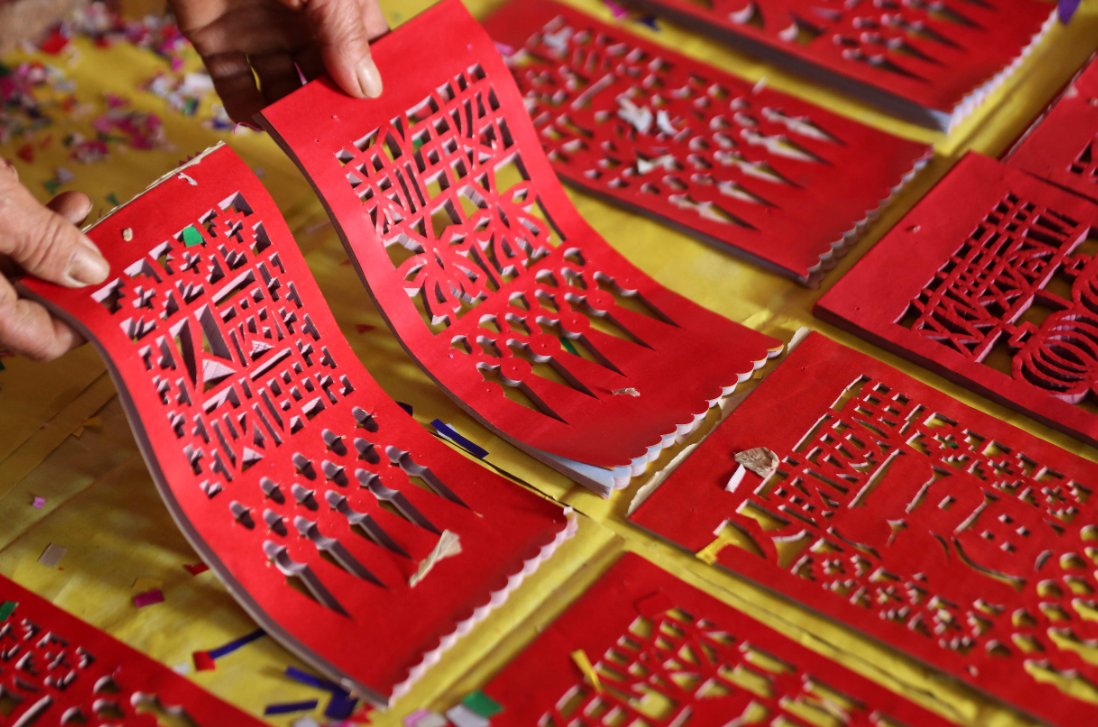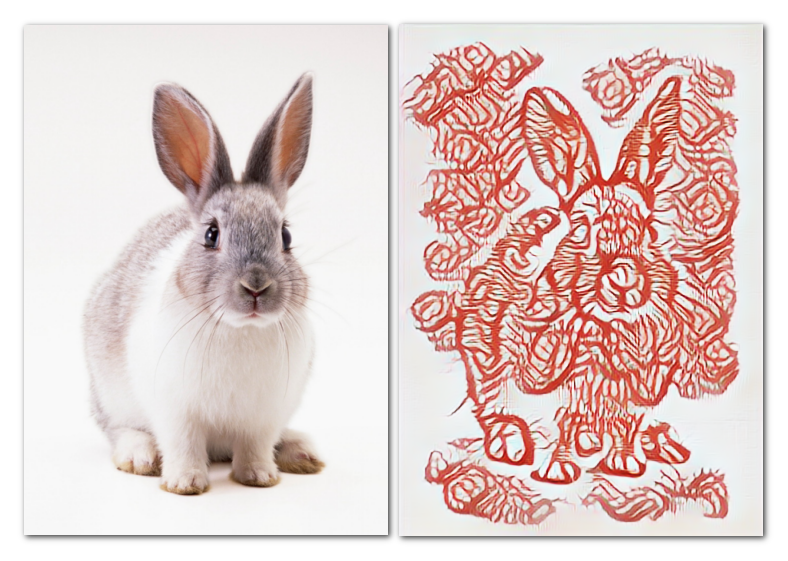### 3.3 烟花兔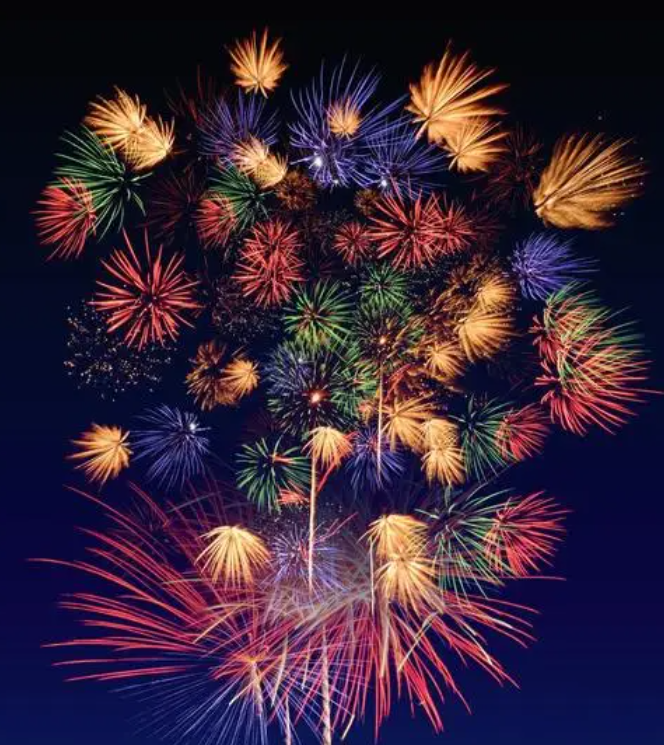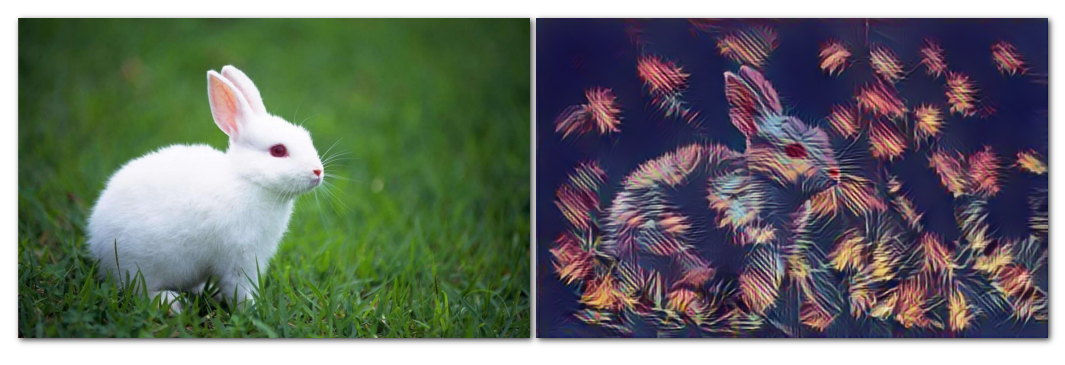## 四、无限遐想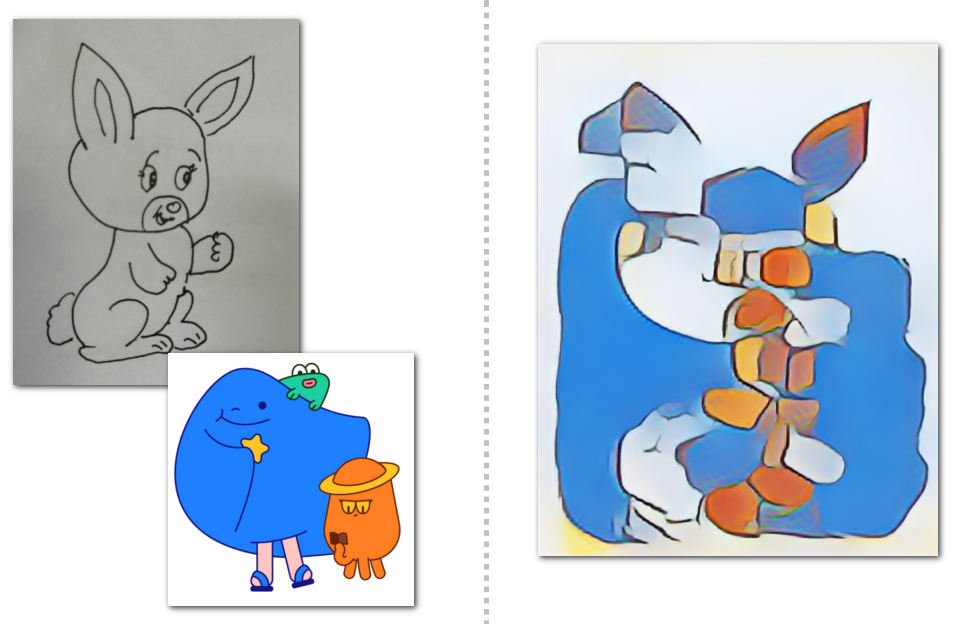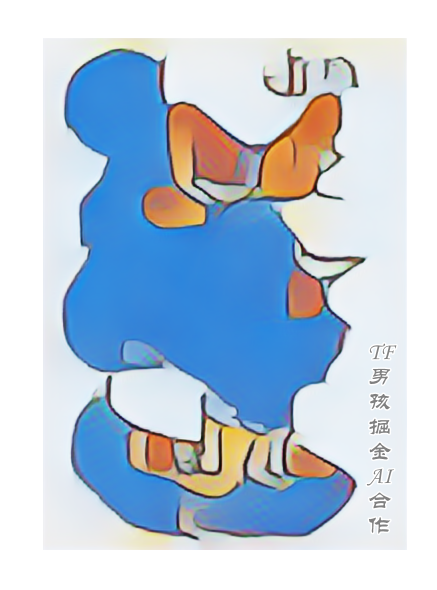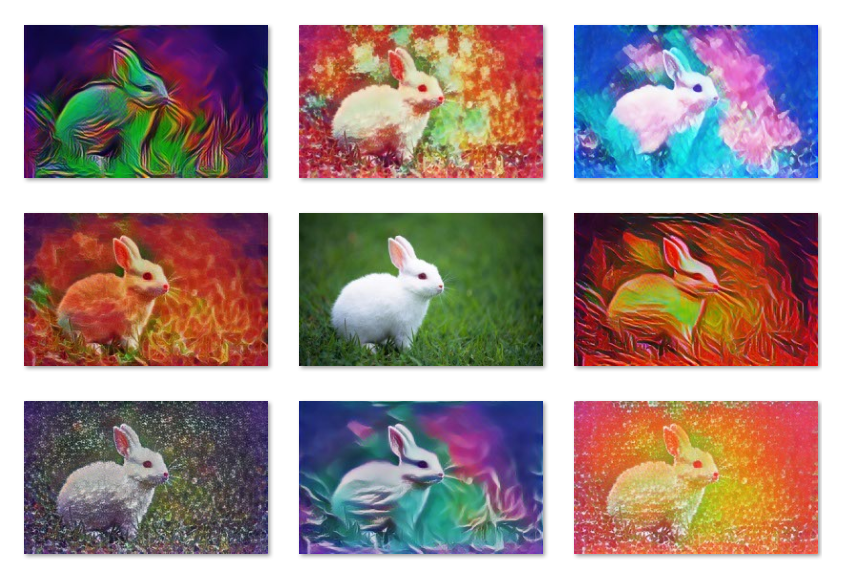「其他文章」